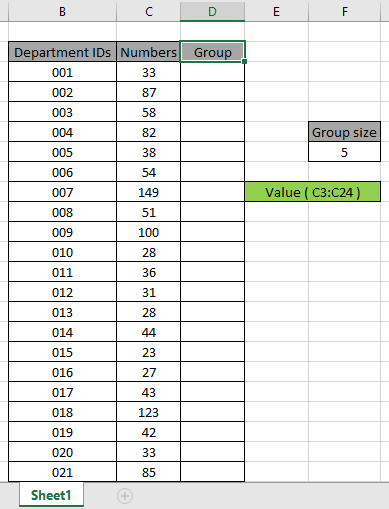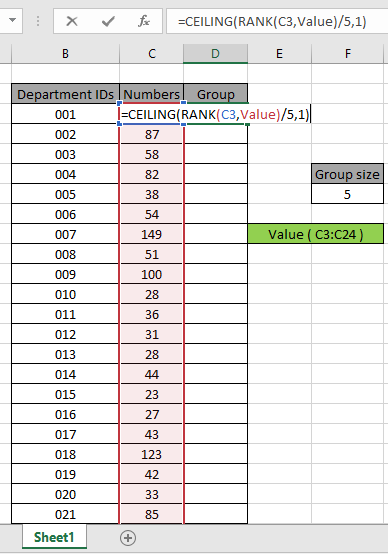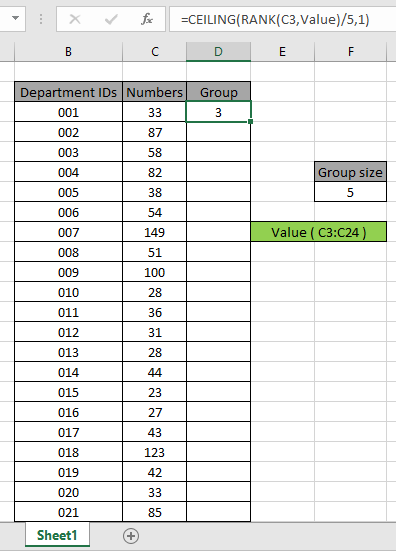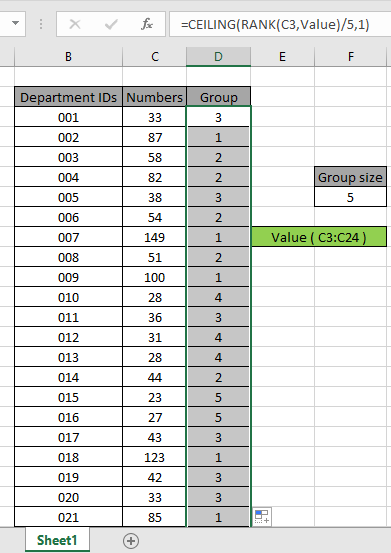# Assign group of size n based on numbers in Excel

In this article, we will learn to return Assign group of size n based on numbers in Excel.

In simple words, while working with a dataset where groups are assigned based on numbers . Sometimes, we need to assign IDs based on numbers in excel. Condition is to get the group of size 5 based on numbers.

How to solve the problem?

For this article we will be needing the use the following functions:

Now we will make a formula out of these functions. Here we will be given a list of values and we need to get their respective group ranked up by numbers.

Use the formula:

= CEILING ( RANK ( number , range ) / size , 1 )

range : list of numbers

number : rank on the basis of number.

size : group size

Example:

All of these might be confusing to understand. So, let's test this formula via running it on the example shown below.

Here we have given data containing numbers. Here we need to find the group number based on the given number and size 5. So for that we have assigned lists in a column for the formula.Now we will use the formula below to get the first number of its group.
Formula:

= CEILING ( RANK ( C3 , Value ) / 5 , 1 )

Explanation:

• RANK ( C3 , Value ) returns the rank as 15.
• The rank is then further divided by size 5 returns 3.
• CEILING function rounds up the number to the nearest integer, if the divide operation returns the decimal numberThe formula must retrurn 3 as the group number to the first value. Here the array to the function is given as named range and cell as cell_reference. Press Enter to get the result.As you can see the formula works fine. Now we need to copy the formula for the other lists. So use the CTRL + D or drag down option of excel.As you can see from the above formula, you can assign a group rank of size 5 based on numbers in Excel using the above explained procedure.

You can also use the ROUNDUP function instead of the CEILING function. ROUNDUP function rounds up the number up to the nearest integer value.
Use the formula:

= ROUNDUP ( RANK ( C3 , Value ) / 5 , 1 )

Here are some observational results using the function

Notes:

1. The formula considers non - numeric values as 0s.
2. The formula considers logic value TRUE as 1 and False as 0.
3. The argument array must be of the same length, otherwise the formula returns error.

Hope this article about how to Assign group of size n based on numbers in Excel is explanatory. Find more articles on SUMPRODUCT functions here. Please share your query below in the comment box. We will assist you.

If you liked our blogs, share it with your friends on Facebook. And also you can follow us on Twitter and Facebook. We would love to hear from you, do let us know how we can improve, complement or innovate our work and make it better for you. Write us at info@exceltip.com

Related Articles

How to use the SUMPRODUCT function in Excel: Returns the SUM after multiplication of values in multiple arrays in excel.

SUM if date is between : Returns the SUM of values between given dates or period in excel.

Sum if date is greater than given date: Returns the SUM of values after the given date or period in excel.

2 Ways to Sum by Month in Excel: Returns the SUM of values within a given specific month in excel.

How to Sum Multiple Columns with Condition: Returns the SUM of values across multiple columns having condition in excel

Popular Articles

50 Excel Shortcut to Increase Your Productivity

Edit a dropdown list

Absolute reference in Excel

If with conditional formatting

If with wildcards

Vlookup by date

Convert Inches To Feet and Inches in Excel 2016

Join first and last name in excel

Count cells which match either A or B

Terms and Conditions of use

The applications/code on this site are distributed as is and without warranties or liability. In no event shall the owner of the copyrights, or the authors of the applications/code be liable for any loss of profit, any problems or any damage resulting from the use or evaluation of the applications/code.